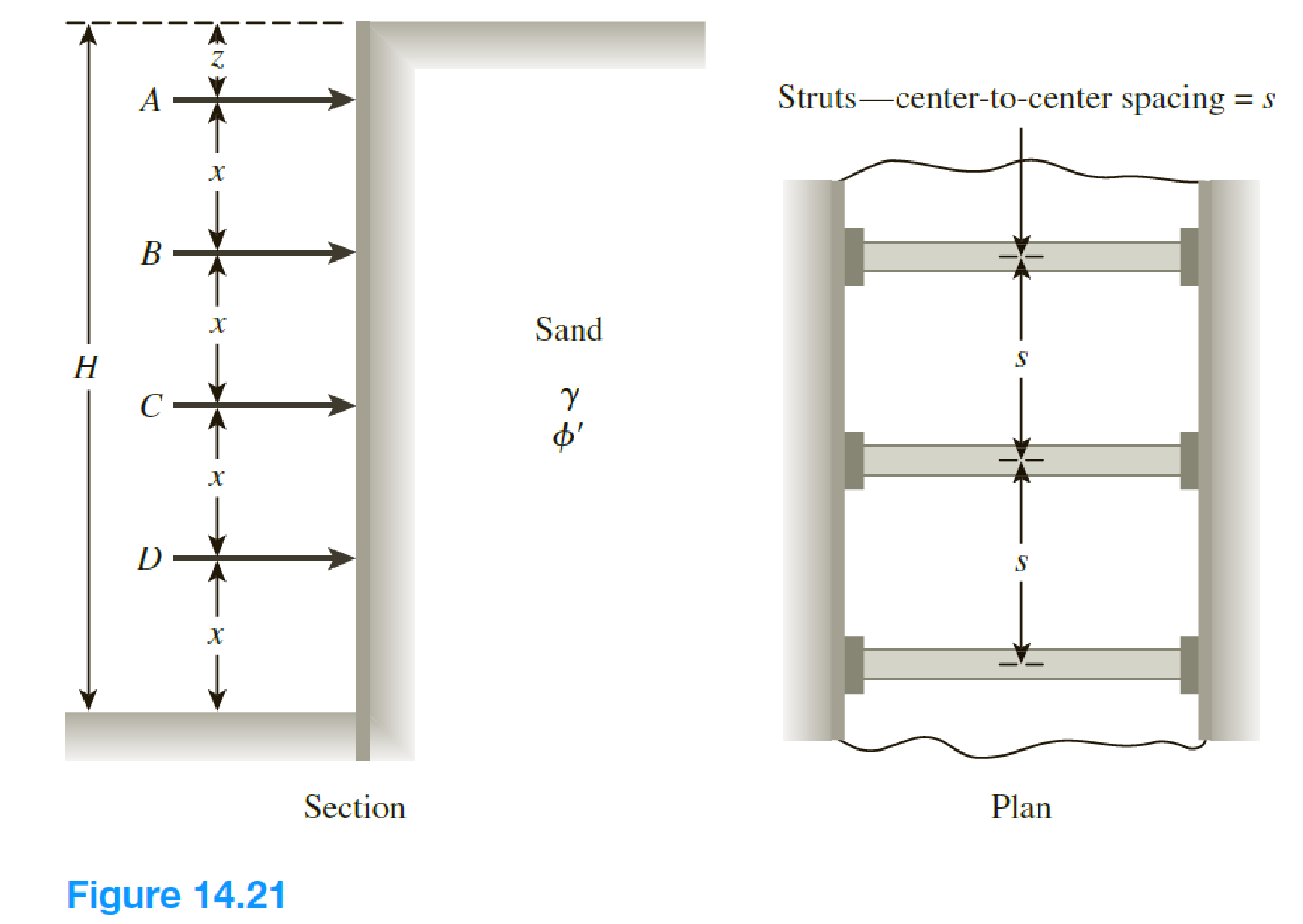Chapter 14, Problem 14.14PPrinciples of Geotechnical Enginee...

9th Edition
Braja M. Das + 1 other
ISBN: 9781305970939

Solutions

Chapter
SectionPrinciples of Geotechnical Enginee...

9th Edition
Braja M. Das + 1 other
ISBN: 9781305970939
Textbook Problem

The elevation and plan of a bracing system for an open cut in sand are shown in Figure 14.21. Using Peck’s empirical pressure diagrams, determine the design strut loads. Given: γsand = 18 kN/m3, ϕ' = 38°, x = 3 m, z = 1.25 m, and s = 3 m.To determine

Find the design strut loads by using the Peck’s empirical pressure.

Explanation

Given information:

The unit weight γsand of the sand is 18kN/m3.

The soil friction angle ϕ is 38°.

The depth z is 1.25 m.

The depth x is 3.0 m.

The depth s is 3.0 m.

Calculation:

Determine the height (H) of the bracing system using the relation.

H=z+x+x+x+s

Substitute 1.25 m for z, 3.0 m for x, and 3.0 m for s.

H=1.25+3.0+3.0+3.0+3.0=13.25m

Determine the maximum pressure intensity σa using the formula.

σa=0.65γHtan2(45ϕ2)

Substitute 18kN/m3 for γ, 13.25 m for H, and 38° for ϕ.

σa=0.65(18)(13.25)tan2(4538°2)=36.9kN/m2

Draw the diagram of level A to B as in Figure 1.

Refer Figure 1.

Determine the reaction at level A by taking moment about level B.

MB1=0A=(13)[(σa×(x+z)((x+z)2))]

Substitute 36.9kN/m2 for σa, 3.0 m for x, and 1.25 m for z.

A=(13)[(36.9×(3+1.25)((3+1.25)2))]=111.08kN/m

Draw the sketch of level B to C as in Figure 2.

Refer Figure 2.

Determine the reaction at level B1 using the relation.

B1=σa(x+z)A

Substitute 36.9kN/m2 for σa, 3.0 m for x, 1.25 m for z, and 111.08kN/m for A.

B1=36.9(3+1.25)111.08=45.75kN/m

Determine the reaction B2 or C1 using the relation.

B2=C1=σa(x)2

Substitute 36.9kN/m2 for σa and 3.0 m for x.

B2=C1=36.9(3.0)2=55.35kN/m

Draw the sketch of level C to D as in Figure 3.

Refer Figure 3.

Determine the reaction at level D by taking moment about level C.

MC2=0D=(13)[σA×(x+s)(x+s2)]

Substitute 36

Still sussing out bartleby?

Check out a sample textbook solution.

See a sample solution

The Solution to Your Study Problems

Bartleby provides explanations to thousands of textbook problems written by our experts, many with advanced degrees!

Get Started

Explain the Kirchhoffs current law.

Engineering Fundamentals: An Introduction to Engineering (MindTap Course List)

How can hole depth be controlled during drilling operations?

Precision Machining Technology (MindTap Course List)

Why must metal that has been used before be cleaned prior to welding?

Welding: Principles and Applications (MindTap Course List)

What other personalization settings do you customize?

Enhanced Discovering Computers 2017 (Shelly Cashman Series) (MindTap Course List)